Conge 精进

2019-01-21

# 01 - Overview

• The focus is on creating a model that can be used to predict future prices for stocks or other assets.
• machine learning provides a suite of tools that support a data-centric way to build predictive models.

Time: 00:00:29

# 02 - The ML problem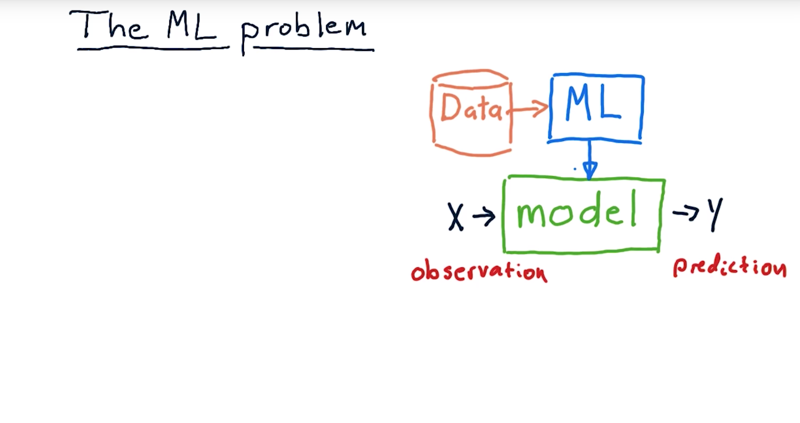The problem the machine learning solves.

1. building a model.
• A model is something that takes in observations like this x here, run it through some sort of process, and provide a y (prediction).
• X can be multidimensional (e.g. Bollinger Bands, PE ratio, and so on).
• Y is typically single-dimension represents the prediction.

Models that don’t use machine learning at all.

• Black-Scholes model that predicts option prices (they develop mathematical formulas).

With machine learning, we’re trying to use data.

• the machine learning process is to take historical data, run it through a machine learning algorithm of some sort to generate the model. Then at runtime or when we need to use the model, we push x’s in it and y’s come out.

Time: 00:01:48

# 03 - quiz Whats X and Y

Now consider you were building a model and we were going to use it in trading in some way.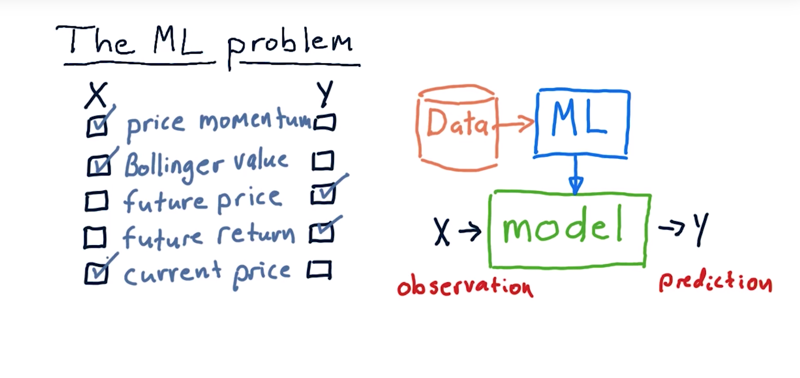# 04 - Supervised regression learning

supervised regression learning.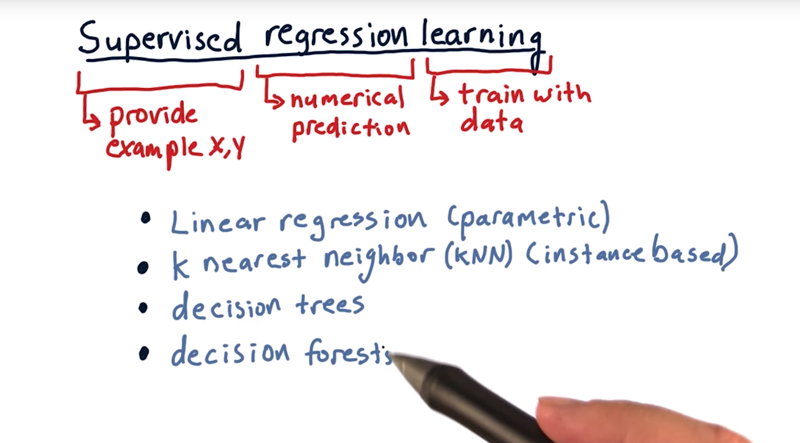• Regression means is we’re trying to make a numerical approximation or a numerical prediction.
• Supervised means that we show the machine the x and also, if you will, the correct answer y.
• learning means training with data.

supervised regression learning techniques:

• linear regression finds parameters for a model. So we call it parametric learning.
• in parametric learning we take the data, munge it around to come up with a few parameters, and then throw the data away.
• K nearest neighbor, or KNN. it is instance based learning.
• In kNN (instance based) we keep all the historical data, the x and y pairs, and when it’s time to make a prediction we consult that data.
• decision trees store a tree structure and when a query comes in, it essentially bounces down that tree according to factors of the data.
• Each node in the tree represents essentially a question, is this x value greater than or less than this other value?
• a leaf and that is the regression value that’s returned.
• Decision forests are simply lots and lots of decision trees taken together, and you query each one to get an overall result.

Time: 00:03:09

# 05 - Robot navigation example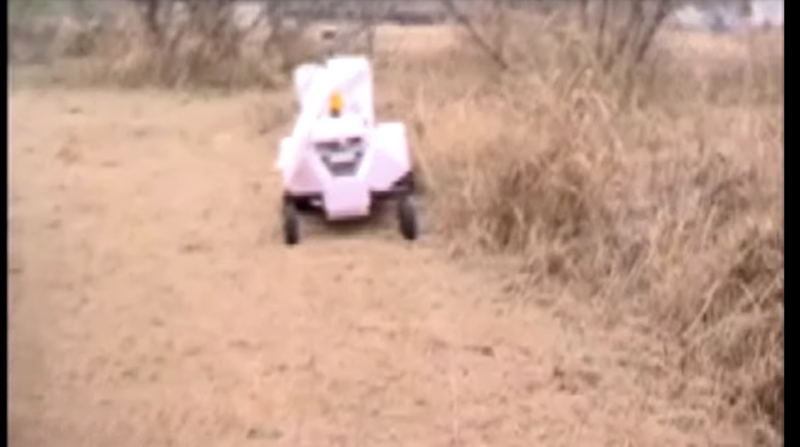Very cool video. Essentially, the robot uses KNN to learn how to navigate.

the input is, in which directions are their obstacles. And also, the robot has the direction towards the goal.

X are these perceptions. Y is, which direction to steer.

Time: 00:03:02

# 06 - How KNN works with stock data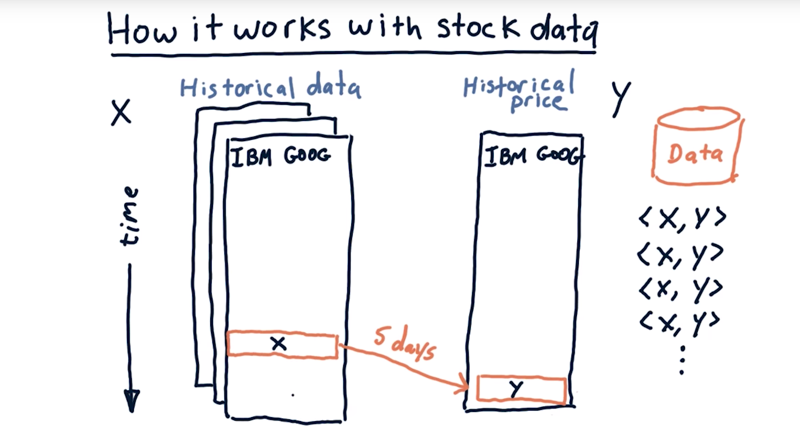• Now we might have many features (X) for each stock, for instance we might have Bollinger Bands, momentum, PE ratio, and so on.
• Y is the historical price.
• We roll back time and look at the values of our features X there, and then look, 5 days into the future to see what that future price (Y) is. We then have an XY pair into our data.
• Then move forward one day get another pair, then another until there are no more Y data.
• now, for each of these days in history, have a pair of X’s and Y’s that we can feed into our database to build our model.

Time: 00:02:19

# 07 - Example at a fintech company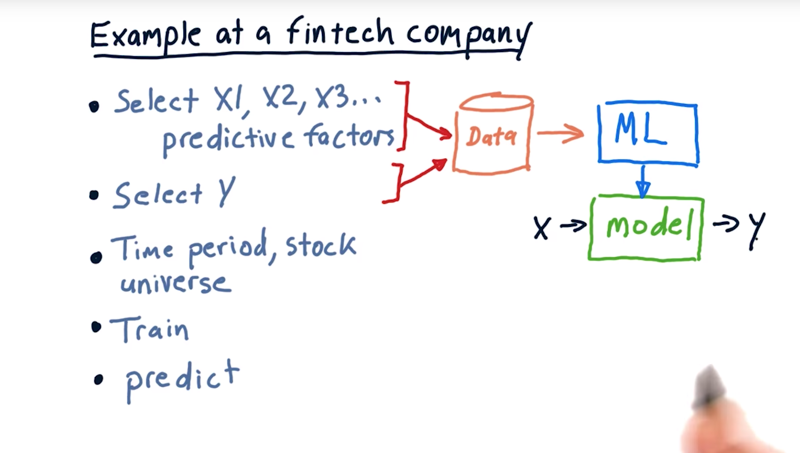The process to build a machine-learning based forecaster at a FinTech company.

1. select which factors do you want to use (Xs). multi-dimensional, Bollinger Bands, PE ratio and so on.
2. select what is it you want to predict (Y): change in price, the market relative change in price, or future price.
3. consider the breadth and depth of the data that we’re going to use to train the system with.
• time period: How far back in time do you want to go to train the system?
• stock universe: What universe of data, which symbols are you going to use to train the system as well?
4. train our model. kNN, a linear regression, decision tree.
5. use that model to do some prediction.
• measure the quantities about the stocks that we want to make a prediction for now (Xs), plug those into the model and the model should provide us our Y, or our prediction.

Time: 00:01:47

# 08 - Price forecasting demo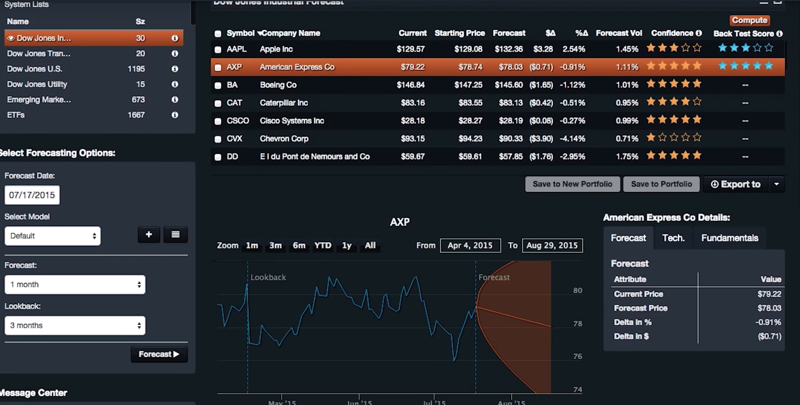• cloud-based application called QuantDesk.

a genetic algorithm for discovering input variables.

• It can do one week forecast. one month forecast and three months of data.

Time: 00:04:01

# 09 - Backtesting

how accurate are these forecasts? back testing: roll back time, and you test your system.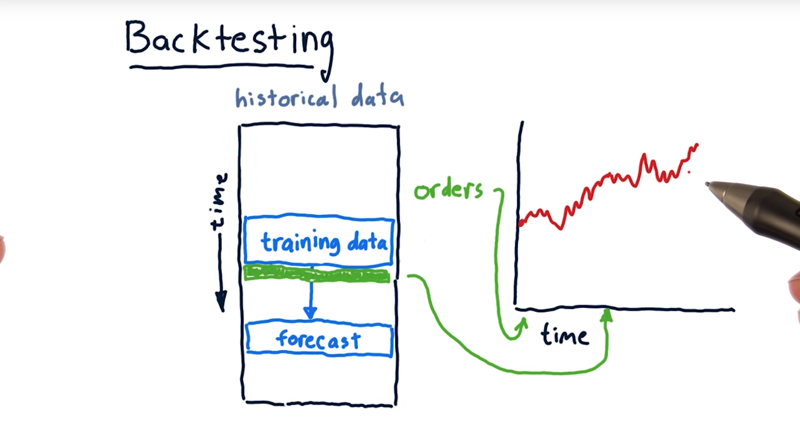So here’s our historical data as usual it’s organized with time coming down.

• limit the data the model sees to a certain amount of time and then make predictions into the simulated future.
• On the basis of that forecast, we can then now place orders, anticipating that that forecast will be achieved.
• then take those orders now, put them into our trading simulator and see how the portfolio works.
• then training over new data, make a new forecast and then make a new set of orders. and enter these orders in our trading simulator and then go forward and see what happens.

And that’s called backtesting.

Time: 00:01:56

# 10 - ML tool in use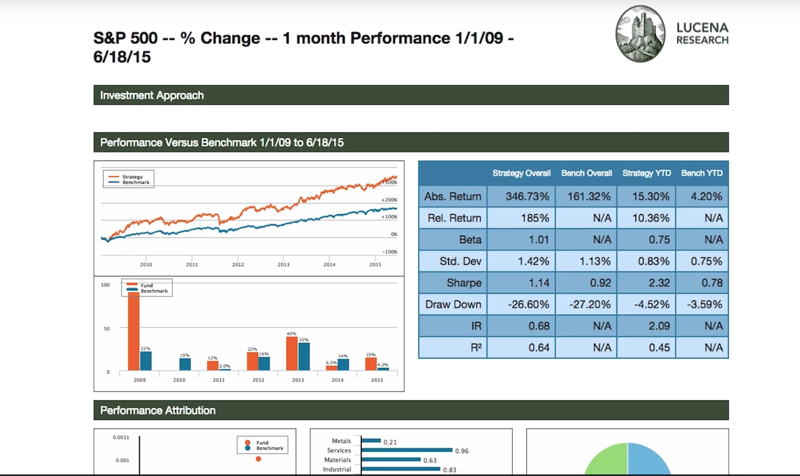that’s the forecast backtest.

Time: 00:01:44

# 11 - Problems with regression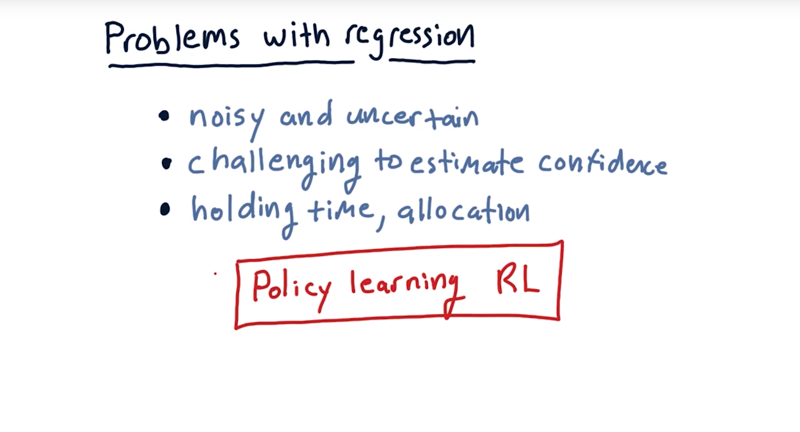challenges using regression based forecasting.

1. our forecasts always seem to be noisy and uncertain.
2. hard to know how confident you should be in a forecast.
• it’s difficult to know how confident you ought to be in any particular forecast.
3. it’s not clear how long you should hold a position that might have arisen from a forecast, and how you should allocate to that position.

Some of these issues can be addressed using reinforcement learning. Where instead of making a forecast of a future price, we had the system learn a policy and the policy tells the system whether to buy or sell a stock.

Time: 00:02:05

# 12 - Problem we will focus on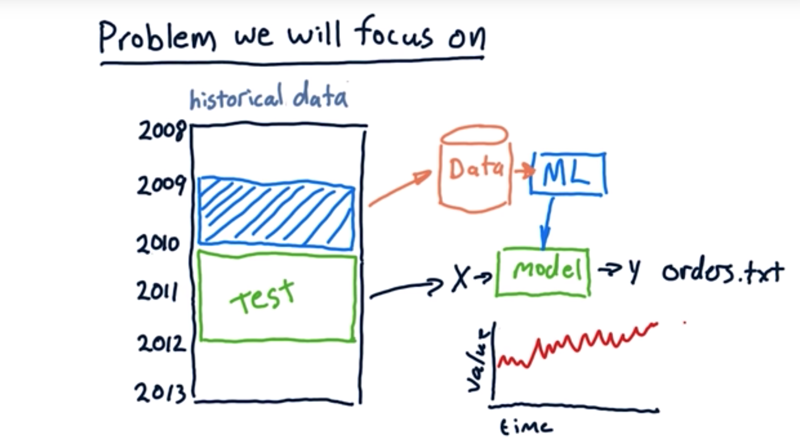1. look at a certain period of data, train our models over that period, and then make forecasts and trade over some other period.
2. our historical data: the period of 2009 as our data to train our model. and test the model with 2010 and 2011 data.
3. using this forecast you’ll generate an orders.txt file which you can push through your market simulator to test how that strategy performs, measure it’s sharp ratio and its total return and so on.
4. compare different machine learning algorithms that generate these orders.

Time: 00:01:18

Total Time: 00:24:18

``````2019-01-21 初稿
``````